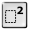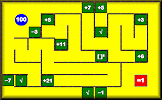# Collecting Like Terms

## Practise your algebraic simplification skills with this self marking exercise.

##### Level 1Level 2DescriptionHelpBracketsMore Algebra

This is level 2; Quadratic expressions.

Simplify each of the following by collecting together like terms.

 $$7x^2 + 3x + 3x^2$$$$7y + 3y^2 - 3y$$$$6w^2 - 4w - 2w^2$$$$3m + 5m^2 + 4m + 9m^2$$$$8n + 5n^2 - 7n + 3n^2$$$$6a - 9a^2 - 5a - 2a^2$$$$9b^2 + 4c + 8b - 4c$$$$5p + 11p^2 + 8p - 6p^2 - 3p$$$$5d^2 - 6e - 3d^2 + 8e - 3d^2$$$$-9c - 6c^2 - 8c - 9c^2$$$$-13k - 9k^2 - 7k - 14k^2$$$$18n^3 + 16n^2 - 18n^3 + 15n$$Check

This is Collecting Like Terms level 2. You can also try:
Collecting Like Terms Level 1 Brackets 1 Brackets 2 Brackets 3 Brackets 4 Brackets 5 Brackets 6 Brackets 7 Brackets 8 Brackets 9

## Instructions

Try your best to answer the questions above. Type your answers into the boxes provided leaving no spaces. As you work through the exercise regularly click the "check" button. If you have any wrong answers, do your best to do corrections but if there is anything you don't understand, please ask your teacher for help.

When you have got all of the questions correct you may want to print out this page and paste it into your exercise book. If you keep your work in an ePortfolio you could take a screen shot of your answers and paste that into your Maths file.

## Transum.org

This web site contains over a thousand free mathematical activities for teachers and pupils. Click here to go to the main page which links to all of the resources available.## More Activities:

Mathematicians are not the people who find Maths easy; they are the people who enjoy how mystifying, puzzling and hard it is. Are you a mathematician?

Comment recorded on the 9 October 'Starter of the Day' page by Mr Jones, Wales:

"I think that having a starter of the day helps improve maths in general. My pupils say they love them!!!"

Comment recorded on the 19 June 'Starter of the Day' page by Nikki Jordan, Braunton School, Devon:

"Excellent. Thank you very much for a fabulous set of starters. I use the 'weekenders' if the daily ones are not quite what I want. Brilliant and much appreciated."

#### BidmazeFind your way through the maze encountering mathematical operations in the correct order to achieve the given total. This is an addictive challenge that begins easy but develops into quite a difficult puzzle.

There are answers to this exercise but they are available in this space to teachers, tutors and parents who have logged in to their Transum subscription on this computer.

A Transum subscription unlocks the answers to the online exercises, quizzes and puzzles. It also provides the teacher with access to quality external links on each of the Transum Topic pages and the facility to add to the collection themselves.

Subscribers can manage class lists, lesson plans and assessment data in the Class Admin application and have access to reports of the Transum Trophies earned by class members.

Subscribe

## Go Maths

Learning and understanding Mathematics, at every level, requires learner engagement. Mathematics is not a spectator sport. Sometimes traditional teaching fails to actively involve students. One way to address the problem is through the use of interactive activities and this web site provides many of those. The Go Maths page is an alphabetical list of free activities designed for students in Secondary/High school.

## Maths Map

Are you looking for something specific? An exercise to supplement the topic you are studying at school at the moment perhaps. Navigate using our Maths Map to find exercises, puzzles and Maths lesson starters grouped by topic.

## Teachers

If you found this activity useful don't forget to record it in your scheme of work or learning management system. The short URL, ready to be copied and pasted, is as follows:

Do you have any comments? It is always useful to receive feedback and helps make this free resource even more useful for those learning Mathematics anywhere in the world. Click here to enter your comments.For All:

© Transum Mathematics :: This activity can be found online at:
www.transum.org/Maths/Activity/Algebra/Collecting_Like_Terms.asp?Level=2

## Description of Levels

CloseLevel 1 - Collect like terms in linear expressions

Example $$5n+4−4n+8$$

Level 2 - Collect like terms in quadratic expressions

Example $$6y+9y^2−3y$$

Brackets Level 1 - Collect like terms when 1 term is repeated

Example: $$5d - (2d + 2)$$

Brackets Level 2 - Collecting like terms when 2 terms are repeated

Example: $$(9d - 7) - (5d - 2)$$

Brackets Level 3 - Multiplying a single positive integer over a bracket

Example: $$4(2d + 4)$$

Brackets Level 4 - Multiplying a single negative integer over a bracket

Example: $$-5(5d + 4)$$

Brackets Level 5 - Multiplying a variable over a bracket

Example: $$4d(5d - 5)$$

Brackets Level 6 - Expanding products of two simple binomials

Example: $$(d + 7)(d - 2)$$

Brackets Level 7 - Expanding products of two binomials

Example: $$(4d + 7)(3d + 2)$$

Brackets Level 8 - Squaring a binomial

Example: $$(3d + 2)^2$$

Brackets Level 9 - Simplifying more complex expressions involving brackets

Example: $$5(3d+1)-2(1-2d)$$

## Collecting Like Terms

This video is from Hegarty Maths.

###### Don't wait until you have finished the exercise before you click on the 'Check' button. Click it often as you work through the questions to see if you are answering them correctly.

Close